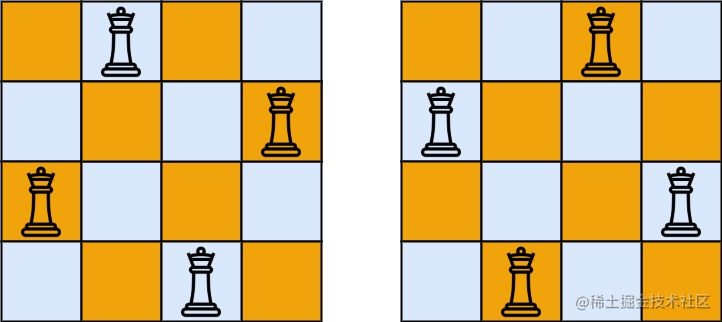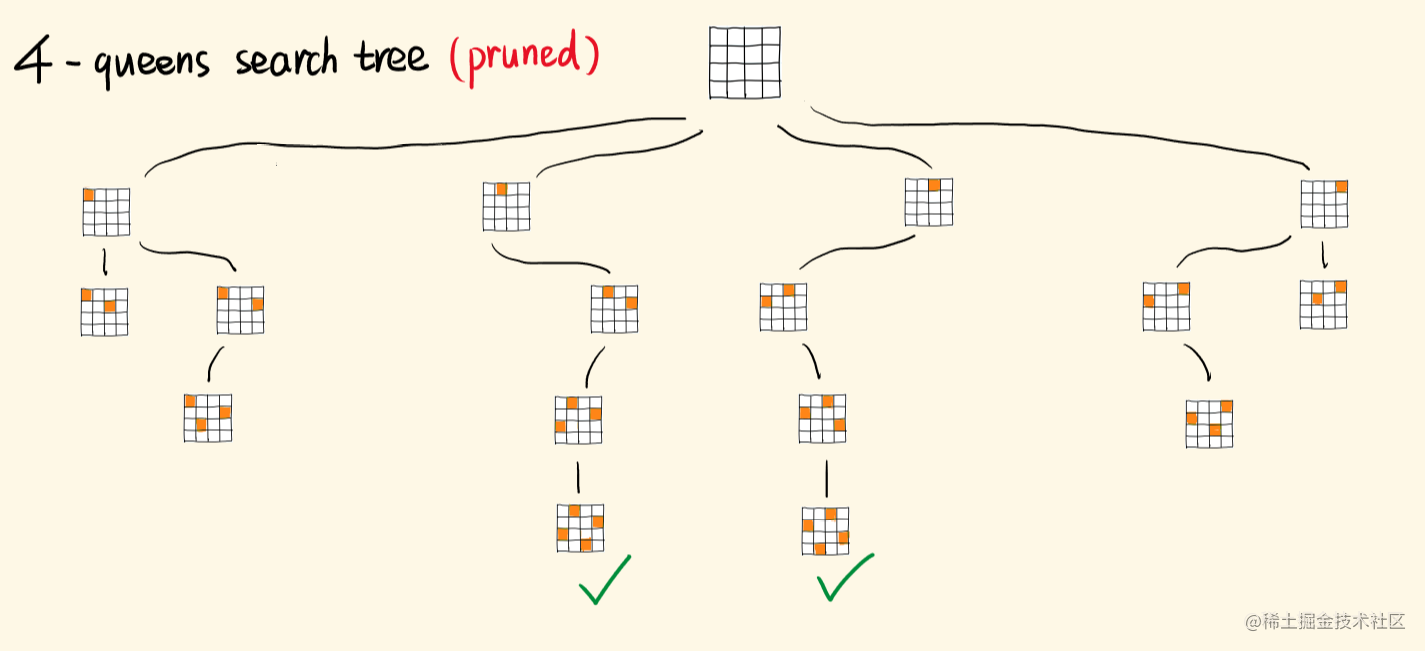# 前端算法面试必刷题系列

## 95. N 皇后 (n-queens)

• DFS + 回溯
• 困难

### 题目

leetcode 传送门``````输入：n = 4

### 基本思路``````a00 a01 a02 a03            0 -1 -2 -3           0  1  2  3
a10 a11 a12 a13    i-j     1  0 -1 -2    i+j    1  2  3  4
a20 a21 a22 a23            2  1  0 -1           2  3  4  5
a30 a31 a32 a33            3  2  1  0           3  4  5  6

### 写法实现

``````const solveNQueens = (n) => {
let res = []
// 棋盘初始化，每个位置暂时都为空 就是 '.'
const board = new Array(n).fill(0).map(item => new Array(n).fill('.'))
// 判断是否合法位置函数，保证皇后之间不冲突
const isValidPosition = (row, col) => {
// 之前每一行都要扫描
for (let i = 0; i < row; i++) {
// 遍历所有的列
for (let j = 0; j < n; j++) {
// 如果该位置有 Q 且 同列 或 在对角线上, 则不合法
// 同行 在这里不判断 是因为我们是一行行来放，所以不会同行
if (board[i][j] === 'Q'
&& (j === col || i + j === row + col || i - j === row - col)) {
return false;
}
}
}
return true;
}
// 又到了我们的 DFS + 回溯环节
const dfs = (row) => {
// 放置当前行
if (row === n) {
// 递归出口 已经超过最后一行，保存当前盘
let boardBackup = board.slice()
let tempRes = boardBackup.map(item => item.join(''))
res.push(tempRes);
return
}
// 开始遍历这一行的每一列的位置
for (let col = 0; col < n; col++) {
// 如果是合法位置，就放下 Q
if (isValidPosition(row, col)) {
board[row][col] = "Q";
// 递归 DFS 下一行
dfs(row + 1);
// 回溯，撤销选择
board[row][col] = '.';
}
}
}
// 从 0 行开始
dfs(0)
return res
}

console.log(solveNQueens(4))

### 优化实现

``````const solveNQueens = (n) => {
let res = []
// 棋盘初始化，每个位置暂时都为空 就是 '.'
const board = new Array(n).fill(0).map(item => new Array(n).fill('.'))
// 判断是否合法位置函数，保证皇后之间不冲突

// 记录已经被占据的列，对角线集合
const occupiedCols = new Set() // 列
const occupiedDiags = new Set() // 对角线
const occupiedReverseDiags = new Set() // 反对角线

// 又到了我们的 DFS + 回溯环节
const dfs = (row) => {
// 放置当前行
if (row === n) {
// 递归出口 已经超过最后一行，保存当前盘
let boardBackup = board.slice()
let tempRes = boardBackup.map(item => item.join(''))
res.push(tempRes);
return
}
// 开始遍历这一行的每一列的位置
for (let col = 0; col < n; col++) {
// 如果当前点的所在的列，所在的对角线都没有皇后，即可选择，否则，跳过这行
if (!occupiedCols.has(col)
&& !occupiedDiags.has(row - col)
&& !occupiedReverseDiags.has(row + col)) {
// 可放置 Q
board[row][col] = 'Q';
// 进行下一行
dfs(row + 1)
// 回溯
board[row][col] = '.';
occupiedCols.delete(col)
occupiedDiags.delete(row - col)
occupiedReverseDiags.delete(row + col)
}
}
}
// 从 0 行开始
dfs(0)
return res
}

console.log(solveNQueens(4))